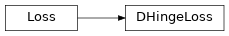# DHingeLoss¶

Inheritance Diagramclass ashpy.keras.losses.DHingeLoss[source]

Bases: tensorflow.python.keras.losses.Loss

Discriminator Hinge Loss as Keras Metric.

See Geometric GAN  for more details.

The Discriminator Hinge loss is the hinge version of the adversarial loss. The Hinge loss is defined as:

$L_{\text{hinge}} = \max(0, 1 -t y)$

where y is the Discriminator output and t is the target class (+1 or -1 in the case of binary classification).

For the case of GANs:

$L_{D_{\text{hinge}}} = - \mathbb{E}_{(x,y) \sim p_data} [ \min(0, -1+D(x,y)) ] - \mathbb{E}_{x \sim p_x, y \sim p_data} [ \min(0, -1 - D(G(z),y)) ]$
  Geometric GAN https://arxiv.org/abs/1705.02894

Methods

 __init__() Initialize the Loss. call(d_real, d_fake) Compute the hinge loss.

Attributes

 reduction Return the current reduction for this type of loss.
__init__()[source]

Initialize the Loss.

Return type: None
call(d_real, d_fake)[source]

Compute the hinge loss.

Return type: Tensor
reduction

Return the current reduction for this type of loss.

Return type: ReductionV2